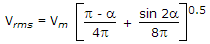# Electronics and Communication Engineering - Power Electronics - Discussion

### Discussion :: Power Electronics - Section 1 (Q.No.21)

21.

A two winding transformer is feeding a single phase half wave rectifier circuit. The load is purely resistive. The rms value of transformer secondary current is Is and rms value of load current in Irms. Then

 [A]. Is = Irms irrespective of the value of firing angle [B]. Is = 0.5 Irms irrespective of the value of firing angle [C]. Is = Irms if firing angle is less than 30° [D]. Is = Irms if firing angle is more than 30°

Explanation:.

 Roger said: (Jul 9, 2021) Explain the formula.